International
Tables for
Crystallography
Volume A
Space-group symmetry
Edited by M. I. Aroyo

International Tables for Crystallography (2016). Vol. A, ch. 1.6, pp. 125-126

## Section 1.6.5.1.2. Status of centrosymmetry and resonant scattering

H. D. Flackb

#### 1.6.5.1.2. Status of centrosymmetry and resonant scattering

| top | pdf |

The basic starting point in this analysis is the following linear transformation ofand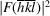, applicable to both observed and model values, to give the average (A) and difference (D) intensities: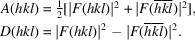In equation (1.6.2.1),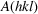was denoted by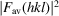. The expression for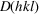corresponding to that forgiven in equation (1.6.2.1)and using the same nomenclature is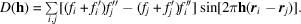In general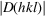is small compared to. A compound with an appreciable resonant-scattering contribution has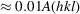, whereas a compound with a small resonant-scattering contribution has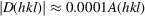. For centric reflections,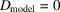, and so the values of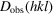of these are entirely due to random uncertainties and systematic errors in the intensity measurements.of acentric reflections contains contributions both from the random uncertainties and the systematic errors of the data measurements, and from the differences betweenandwhich arise through the effect of resonant scattering. A slight experimental limitation is that a data set of intensities needs to contain both reflections hkl and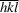in order to obtain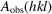and.

The Bijvoet ratio, defined by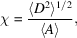is the ratio of the root-mean-square value of D to the mean value of A. In a structure analysis, two independent estimates of the Bijvoet ratio are available and their comparison leads to useful information as to whether the crystal structure is centrosymmetric or not.

The first estimate arises from considerations of intensity statistics leading to the definition of the Bijvoet ratio as a value called Friedifstat, whose functional form was derived by Flack & Shmueli (2007) and Shmueli & Flack (2009). One needs only to know the chemical composition of the compound and the wavelength of the X-radiation to calculate Friedifstat using various available software.

The second estimate of the Bijvoet ratio, Friedifobs, is obtained from the observed diffraction intensities. One problematic point in the evaluation of Friedifobs arises because A and D do not have the same dependence on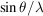and it is necessary to eliminate this difference as far as possible. A second problematic point in the calculation is to make sure that only acentric reflections of any of the noncentrosymmetric point groups in the chosen Laue class are selected for the calculation of Friedifobs. In this way one is sure that if the point group of the crystal is centrosymmetric, all of the chosen reflections are centric, and if the point group of the crystal is noncentrosymmetric, all of the chosen reflections are acentric. The necessary selection is achieved by taking only those reflections that are general in the Laue group. To date (2015), the calculation of Friedifobs is not available in distributed software. On comparison of Friedifstat with Friedifobs, one is able to state with some confidence that:

 (1) if Friedifobs is much lower than Friedifstat, then the crystal structure is either centrosymmetric, and random uncertainties and systematic errors in the data set are minor, or noncentrosymmetric with the crystal twinned by inversion in a proportion close to 50:50; (2) if Friedifobs is close in value to Friedifstat, then the crystal is probably noncentrosymmetric and random uncertainties and systematic errors in the data set are minor. However, data from a centrosymmetric crystal with large random uncertainties and systematic errors may also produce this result; and (3) if Friedifobs is much larger than Friedifstat then either the data set is dominated by random uncertainties and systematic errors or the chemical formula is erroneous.

#### Example 1

The crystal of compound Ex1 (Udupa & Krebs, 1979) is known to be centrosymmetric (space group) and has a significant resonant-scattering contribution, Friedifstat = 498 and Friedifobs = 164. The comparison of Friedifstat and Friedifobs indicates that the crystal structure is centrosymmetric.

#### Example 2

The crystal of compound Ex2, potassium hydrogen (2R,3R) tartrate, is known to be enantiomerically pure and appears in space group. The value of Friedifobs is 217 compared to a Friedifstat value of 174. The agreement is good and allows the deduction that the crystal is neither centrosymmetric, nor twinned by inversion in a proportion near to 50:50, nor that the data set is unsatisfactorily dominated by random uncertainty and systematic error.

#### Example 3

The crystals of compound Ex3 (Zhu & Jiang, 2007) occur in Laue group. One finds Friedifstat = 70 and Friedifobs = 499. The huge discrepency between the two shows that the observed values of D are dominated by random uncertainty and systematic error.

### References

Flack, H. D. & Shmueli, U. (2007). The mean-square Friedel intensity difference in P1 with a centrosymmetric substructure. Acta Cryst. A63, 257–265.
Shmueli, U. & Flack, H. D. (2009). Concise intensity statistics of Friedel opposites and classification of the reflections. Acta Cryst. A65, 322–325.
Udupa, M. R. & Krebs, B. (1979). Crystal and molecular structure of creatininium tetrachlorocuprate(II). Inorg. Chim. Acta, 33, 241–244.
Zhu, H.-Y. & Jiang, S.-D. (2007). 1,3,4,6-Tetra-O-acetyl-2-(trifluoromethylsulfonyl)-β-D-mannopyranose. Acta Cryst. E63, o2833.